Courses

# Interpolation - Interpolation and Extrapolation, Business Mathematics and Statistics B Com Notes | EduRev

## B Com : Interpolation - Interpolation and Extrapolation, Business Mathematics and Statistics B Com Notes | EduRev

The document Interpolation - Interpolation and Extrapolation, Business Mathematics and Statistics B Com Notes | EduRev is a part of the B Com Course Business Mathematics and Statistics.
All you need of B Com at this link: B Com

Interpolation is a useful mathematical and statistical tool used to estimate values between two points. In this lesson, you will learn about this tool, its formula and how to use it.

What Is Interpolation?

Interpolation is the process of finding a value between two points on a line or curve. To help us remember what it means, we should think of the first part of the word, 'inter,' as meaning 'enter,' which reminds us to look 'inside' the data we originally had. This tool, interpolation, is not only useful in statistics, but is also useful in science, business or any time there is a need to predict values that fall within two existing data points.

Interpolation Example

Here's an example that will illustrate the concept of interpolation. A gardener planted a tomato plant and she measured and kept track of its growth every other day. This gardener is a curious person, and she would like to estimate how tall her plant was on the fourth day.

Her table of observations looked like this: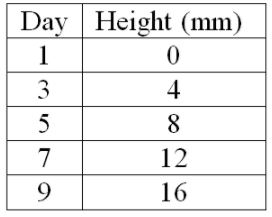Based on the chart, it's not too difficult to figure out that the plant was probably 6 mm tall on the fourth day. This is because this disciplined tomato plant grew in a linear pattern; there was a linear relationship between the number of days measured and the plant's height growth. Linear pattern means the points created a straight line. We could even estimate by plotting the data on a graph.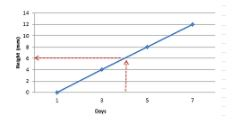But what if the plant was not growing with a convenient linear pattern? What if its growth looked more like this?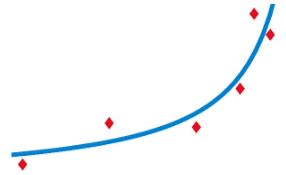What would the gardener do in order to make an estimation based on the above curve? Well, that is  where the interpolation formula would come in handy.

Interpolation Formula

The interpolation formula looks like this: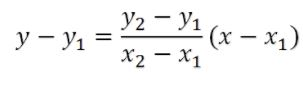Now, let's explore how to use this formula. The two sets of points between which the estimate can be found are: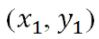Going back to the tomato plant example, the first set of values for day three are (3,4), the second set of values for day five are (5,8), and the value for x is 4 since we want to find the height, y, on the fourth day. After substituting these values into the formula, calculate the estimated height of the plant on the fourth day.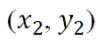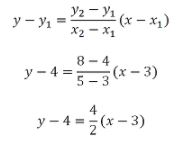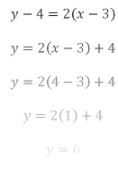Based on the calculations, the estimated height of the plant on the fourth day is 6 mm.

Offer running on EduRev: Apply code STAYHOME200 to get INR 200 off on our premium plan EduRev Infinity!

122 videos|142 docs

,

,

,

,

,

,

,

,

,

,

,

,

,

,

,

,

,

,

,

,

,

,

,

,

;